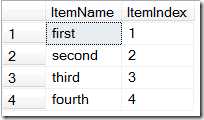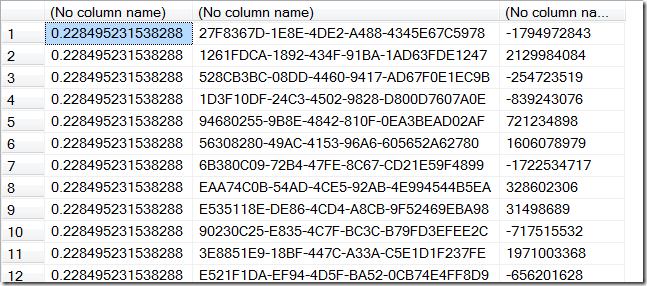Philip Hendry's Blog

# Numbering XML Elements in T-SQL

Jan 5, 2010 • T-SQL • 1 min read

I had a problem where I wanted to convert a chunk of XML passed to a T-SQL stored procedure into a relational model but at the same time number the elements. I used row_number() but that required an order by clause and I actually wanted to maintain the original order of the elements and not apply any other ordering. Initially I tried adding order by 1 to try and fool it into apply ‘no order’ but that didn’t work, instead I replaced the 1 with rand(). Because rand() is not recomputed for each row it effectively orders by a single number which is the equivalent of order by 1. Here’s the code :

``````declare @xml xml
set @xml = '
<root>
<item>first</item>
<item>second</item>
<item>third</item>
<item>fourth</item>
</root>'

select
T.c.value('.', 'nvarchar(25)') as ItemName,
row_number() over (order by rand()) as ItemIndex
from @xml.nodes('/root/item') as T(c)
``````

Which returns :The use of the rand() function is a little strange but the code below should highlight what it’s doing :

``````select rand(), newid(), checksum(newid())
from master..spt_values
where [type] = 'P'
``````

Which returns :As you can see the rand() returns the same number for every row since it is computed at the start of the query execution. However, newid() is recomputed for every row and by wrapping it with checksum() you can also use it to calculate random numbers for every row.

Post by: Philip Hendry